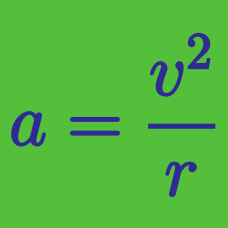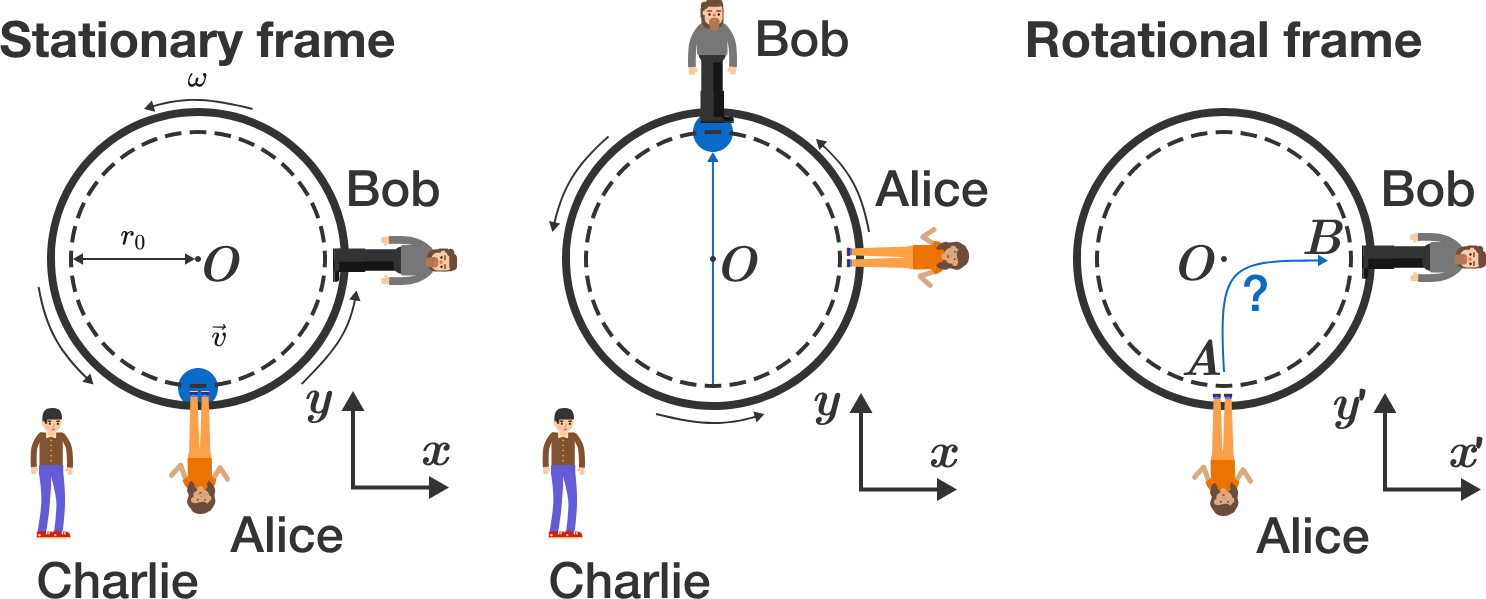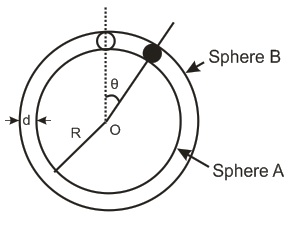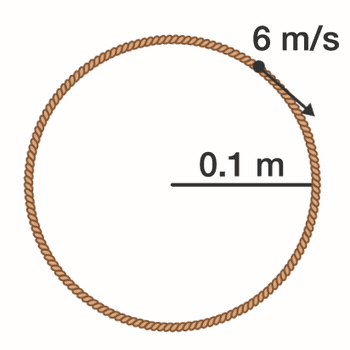Classical Mechanics

# Circular Motion - Level 4 Challenges

Alice and Bob are having fun throwing a ball to each other on a merry-go-round. Charlie looks at the game from outside of the merry-go-round. From his perspective, the ball thrown by Alice flies straight along the $y$-axis at a constant velocity of $\vec v = v_0 \vec e_y$ to Bob. Bob can catch this ball after the flight time $t_0 = T/4,$ because the merry-go-round has completed a quarter turn in the meantime.But how does Alice observe (rotating reference system)? What average speed (average of absolute value of velocity vector) $\overline{v'} = \frac{1}{t_0} \int_0^{t_0} |\vec v\,'| dt$ does the ball have from Alice's perspective?

Give the answer in units of $v_0$ and with an accuracy of 3 decimal places.


Hints:

• The merry-go-round rotates with constant frequency $\omega = 2 \pi/T$, so that Alice $($point $r_A)$ and Bob $($point $r_B)$ move on circular paths in the stationary reference system. In the rotating reference system, both points $r_A^\prime$ and $r_B^\prime$ are stationary.
• Search for a $2\times 2$ matrix $\mathbf{D}$ such that the transformation reads $\vec{r}^\prime = \mathbf{D} \cdot \vec{r}$. For the calculation of the average velocity, you may use the integral: $\int \sqrt{1 + x^2} d x = \frac{1}{2} \left( x \sqrt{1 + x^2} + \text{arcsinh}(x) \right).$

Bonus question: Which (fictitious) forces act on the ball in the rotating reference system? How can we explain the path of the ball?A spherical ball of mass $m$ is kept at the highest point in the space between two fixed concentric spheres $A$ and $B$ . The smaller sphere $A$ has a radius $R$ and the space between the two spheres has a width $d$. The ball has a diameter very slightly less than $d$ . All surfaces are frictionless. The ball is given a gentle push towards the right . The angle made by the radius vector of the ball with the upward vertical is $\theta$. What is the total normal reaction force exerted by the spheres on the ball in terms of angle $\theta$?A rope loop of radius $r = \SI[per-mode=symbol]{0.1}{\meter}$ and mass $m = 50\pi\,\text{g}$ rotates on a frictionless table such that the tangential velocity of any point on the loop is $v_\textrm{tangential}=\SI[per-mode=symbol]{6}{\meter\per\second}.$

Find the tension in the loop (in Newtons).

A boy runs on a circular path of radius $R=28\text{ meters}$ with constant speed $u=4 \text{ m/s}$.

Another boy starts from the center of the circle to catch the first boy. The second boy always moves towards the first one. and maintains a speed of $v = 4\text{ m/s}$. How long will the chase last in seconds?

Details and Assumptions:

1) Ignore air friction.

2) Take $\pi = \dfrac{22}7$.

Consider a body, attached to a string of length $r$, to be initially at rest in the vertical plane. It can be proved that if we give the body an initial velocity of $v>\sqrt{5gr}$, then, the body can make complete revolutions in the vertical plane about the point of suspension.

Let us consider that the body, initially at rest, is given a velocity $v<\sqrt{5gr}$. If at this value of $v$ the body eventually hits the point of suspension then we can write:

$v^2 = (a+\sqrt{b})gr \; .$

Find $a\times b$.

×